# Solving a system of two simultaneous trigonometric equations

#### Jeroen Staps

1. The problem statement, all variables and given/known data
I need to solve a system of two equations for T and θ algebraic and with all the other parameters known.
φ is equal to: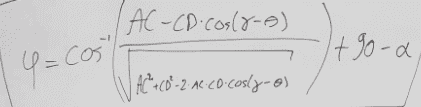2. Relevant equations
The relevant equations are the two equations left of * in the image below

3. The attempt at a solution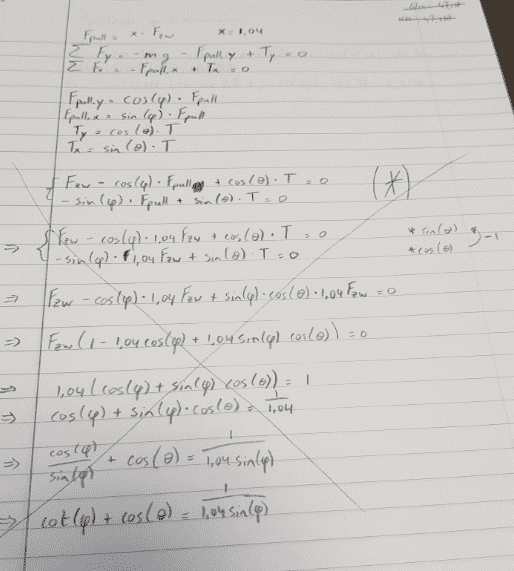I tried Gauss elimination but I could not solve it further.

Now my question is if it is even possible to solve this algebraic to find T and θ.

#### Attachments

• 340 KB Views: 105
• 26 KB Views: 113

#### kuruman

Homework Helper
Gold Member
You have a system of two equations and two unknowns. It should be solvable. Write
$T\cos\theta=F_{pull}\cos\phi-F_w$
$T\sin\theta=F_{pull}\sin\phi$
Square both sides of each equation and add them. This eliminates $\theta$. Solve for $T$. Then divide the bottom equation by the top to eliminate $T$. Find $\tan\theta$ and hence $\theta$.

#### Jeroen Staps

You have a system of two equations and two unknowns. It should be solvable. Write
$T\cos\theta=F_{pull}\cos\phi-F_w$
$T\sin\theta=F_{pull}\sin\phi$
Square both sides of each equation and add them. This eliminates $\theta$. Solve for $T$. Then divide the bottom equation by the top to eliminate $T$. Find $\tan\theta$ and hence $\theta$.
Then I get this: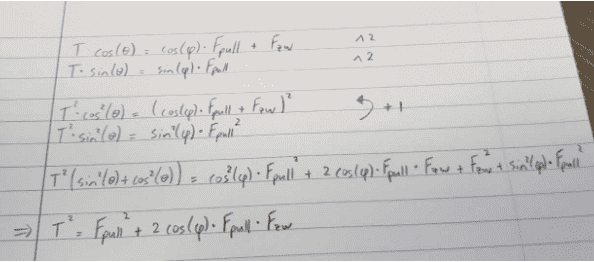And φ still contains θ in it

#### Attachments

• 96.9 KB Views: 105

#### kuruman

Homework Helper
Gold Member
Then I get this:View attachment 240241

And φ still contains θ in it
Oops, I missed that. The expression for $\phi$ can be written as
$\sin(\phi+\alpha)=\frac{(AC)-(CD)\cos(\gamma-\theta)}{\sqrt{(AC)^2+(CD)^2-2(AC)(CD)\cos(\gamma-\theta)}}$
You also know that
$\tan\theta=\frac{F_{pull}\sin\phi}{F_{pull}\cos\phi-F_w}$
In principle you can expand the trigonometric functions of sums of angles using the known trig identities and then substitute
$\cos\phi=x ~~\rightarrow~\sin\phi=\sqrt{1-x^2}$
$\cos\theta=y ~~\rightarrow~\sin\theta=\sqrt{1-y^2}$
This should give you a system of two equations and two unknowns, but it's not going to be a pretty picture. I would check my derivation for $\phi$ and see if I missed something that might simplify it. You never know ...

#### Jeroen Staps

Oops, I missed that. The expression for $\phi$ can be written as
$\sin(\phi+\alpha)=\frac{(AC)-(CD)\cos(\gamma-\theta)}{\sqrt{(AC)^2+(CD)^2-2(AC)(CD)\cos(\gamma-\theta)}}$
You also know that
$\tan\theta=\frac{F_{pull}\sin\phi}{F_{pull}\cos\phi-F_w}$
In principle you can expand the trigonometric functions of sums of angles using the known trig identities and then substitute
$\cos\phi=x ~~\rightarrow~\sin\phi=\sqrt{1-x^2}$
$\cos\theta=y ~~\rightarrow~\sin\theta=\sqrt{1-y^2}$
This should give you a system of two equations and two unknowns, but it's not going to be a pretty picture. I would check my derivation for $\phi$ and see if I missed something that might simplify it. You never know ...
The problem is that I keep getting a inverse cosine inside a cosine or a sine and when I get this there is no possibility to get θ out of the inverse cosine right?

#### kuruman

Homework Helper
Gold Member
The problem is that I keep getting a inverse cosine inside a cosine or a sine and when I get this there is no possibility to get θ out of the inverse cosine right?
I am not sure I understand what you mean. You can write, for example, $\sin(\phi+\alpha)=\cos(\alpha) \sqrt{1-x^2}+\sin(\alpha)x$, $\cos(\gamma-\theta)=\cos(\gamma)y+\sin(\gamma)\sqrt{1-y^2}$ and so on. You solve for $x$ and $y$ to get the cosines in terms of known quantities. Once you have these, there is only one inverse cosine to find for each if it's angles that you are required to have.

Incidentally, you should never have to consider an inverse trig function inside another trig function. For example, say you have $\theta=\arcsin(y/x)$. Clearly $\sin(\theta) = y/x$. What is $\cos(\theta)$? Remember that $\sin(\theta)=\frac{opp.}{hyp.}$ and $\cos(\theta)=\frac{adj.}{hyp.}$. Identify $opp. = y$ and $hyp. = x$ which makes $adj. = \sqrt{x^2-y^2}$ and $\cos(\theta)=\frac{\sqrt{x^2-y^2}}{x}$. It follows that $\tan(\theta)=\frac{y}{\sqrt{x^2-y^2}}$.

#### vela

Staff Emeritus
Homework Helper
If you draw the three vectors tail-to-tip, they'll form a triangle. You can use the law of sines and cosines to try find an expression for $\theta$.

#### SammyS

Staff Emeritus
Homework Helper
Gold Member
The problem is that I keep getting a inverse cosine inside a cosine or a sine and when I get this there is no possibility to get θ out of the inverse cosine right?
I think that in order to get more help, you need to give more detail regarding the overall problem you are solving. That may take this from being mainly a problem with algebra and trig, to a physics or mechanical engineering problem.

In particular, that initial equation you give looks like it comes from having some constraints on the directions of the tension, $\ \vec T \,,\$ and the pulling force, $\vec{F}_\text{pull}\,.$ These constraints appear to be related to the positions of three points, A, C, and D along with two angles, $\ \alpha \$ and $\ \gamma \,.$

The denominator of the following appears to be from the application of the Law of Cosines for triangle ACD and may simply be the length AD .

$\sin(\phi+\alpha)=\dfrac{(AC)-(CD)\cos(\gamma-\theta)}{\sqrt{(AC)^2+(CD)^2-2(AC)(CD)\cos(\gamma-\theta)}}$

#### SammyS

Staff Emeritus
Homework Helper
Gold Member
From the thread by @Jeroen Staps , Post #46 : https://www.physicsforums.com/threads/solving-a-system-of-two-simultaneous-trigonometric-equations.968051/
View attachment 239863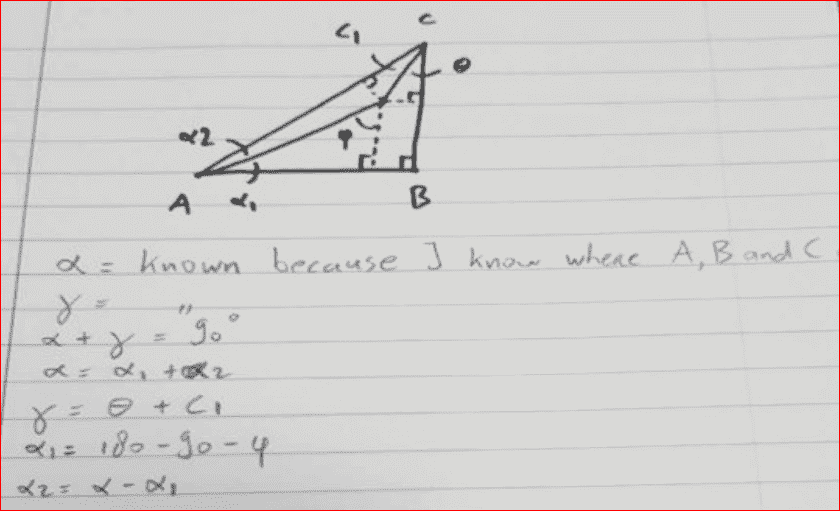Need more?
And from Post #31 of the above referenced thread: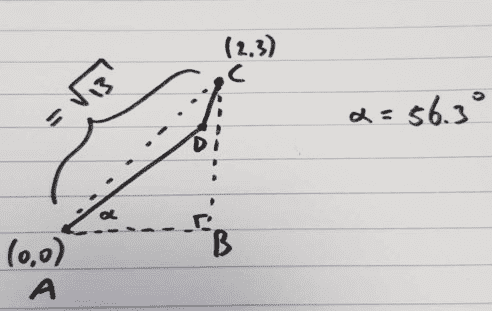Also, m∠ACB = $\gamma \,.$

For one thing $\alpha + \gamma =$ 90° .

@Jeroen Staps : Do you know the length CD? I see that from the other thread it's length is fixed, but the length AD is not fixed.

#### Attachments

• 211.4 KB Views: 44
• 110.7 KB Views: 45
Last edited:

#### Jeroen Staps

Do you know the length CD? I see that from the other thread it's length is fixed, but the length AD is not fixed
Yes, CD is fixed and AD is not.
And the length of CD is known

Last edited:

#### SammyS

Staff Emeritus
Homework Helper
Gold Member
Yes, CD is fixed and AD is not.
And the length of CD is known
Are the coordinates of points A, B, and C the same as given in your figure, or are those simply for example?

If those are the values to be used, then what is the length CD ?

By the way, there is a relatively straight forward expression for $\ \tan(\varphi)\$ in terms of $\ \sin(\theta) \$ and $\ \cos(\theta) \$ together with the lengths of AB, BC, and CD . This expression does not refer to either of the angles, $\alpha$, or $\gamma \,.$

#### Jeroen Staps

Are the coordinates of points A, B, and C the same as given in your figure, or are those simply for example?

If those are the values to be used, then what is the length CD ?

By the way, there is a relatively straight forward expression for $\ \tan(\varphi)\$ in terms of $\ \sin(\theta) \$ and $\ \cos(\theta) \$ together with the lengths of AB, BC, and CD . This expression does not refer to either of the angles, $\alpha$, or $\gamma \,.$
The coordinates are for example as is the length of CD. The goal is to express φ in terms of θ or the other way around. And all the other parameters in the expression have to be known.

#### SammyS

Staff Emeritus
Homework Helper
Gold Member
The coordinates are for example as is the length of CD. The goal is to express φ in terms of θ or the other way around. And all the other parameters in the expression have to be known.
Thanks for the clarifications.

Now that we have point B established in this thread, the following expression relates $\varphi$ and $\theta$.

$\tan(\varphi) = \dfrac{AB-CD \sin(\theta)} {BC-CD\cos(\theta}$​

If I can come up with a reasonable, well-labelled figure, I will post the derivation of the above in your other thread: https://www.physicsforums.com/threads/describe-the-position-of-a-pulley-attached-to-a-sling.967018/

#### Jeroen Staps

Thanks for the clarifications.

Now that we have point B established in this thread, the following expression relates $\varphi$ and $\theta$.

$\tan(\varphi) = \dfrac{AB-CD \sin(\theta)} {BC-CD\cos(\theta}$​

If I can come up with a reasonable, well-labelled figure, I will post the derivation of the above in your other thread: https://www.physicsforums.com/threads/describe-the-position-of-a-pulley-attached-to-a-sling.967018/
Is it now possible to extract theta from $\cos(\arctan(\dfrac{AB-CD \sin(\theta)} {BC-CD\cos(\theta)}))$

I know that $\cos(\arctan(x)) =\dfrac{1}{\sqrt{x^2+1}}$

### The Physics Forums Way

We Value Quality
• Topics based on mainstream science
• Proper English grammar and spelling
We Value Civility
• Positive and compassionate attitudes
• Patience while debating
We Value Productivity
• Disciplined to remain on-topic
• Recognition of own weaknesses
• Solo and co-op problem solving